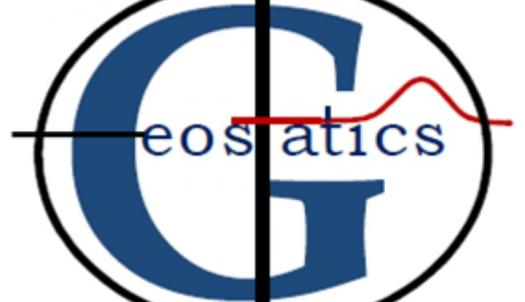# How Well Do You Know Geostatistics?

Approved & Edited by ProProfs Editorial Team
At ProProfs Quizzes, our dedicated in-house team of experts takes pride in their work. With a sharp eye for detail, they meticulously review each quiz. This ensures that every quiz, taken by over 100 million users, meets our standards of accuracy, clarity, and engagement.
| Written by Cripstwick
C
Cripstwick
Community Contributor
Quizzes Created: 693 | Total Attempts: 678,987
Questions: 10 | Attempts: 193SettingsGeostatistics is a branch of statistics that merges with geography. It is applicable to many areas of our life. Take this intelligent quiz to reveal more knowledge of Geostatistics.

• 1.

### Who was the founder of Geostatistics?

• A.

Georges Matheron

• B.

Pythagoras Pierre de Fermat

• C.

Leonhard Euler

• D.

Isaac Newton

A. Georges Matheron
Explanation
Georges Matheron is the correct answer because he is widely recognized as the founder of Geostatistics. He was a French mathematician and engineer who developed the theory and methods of Geostatistics in the 1960s. Matheron's work revolutionized the field of spatial analysis and paved the way for the application of statistical techniques in the study of spatial data. His contributions have had a significant impact on various disciplines, including geology, mining, hydrology, and environmental sciences.

Rate this question:

• 2.

### The founder of Geostatistics lived for how many years?

• A.

70

• B.

69

• C.

68

• D.

71

B. 69
Explanation
The founder of Geostatistics lived for 69 years.

Rate this question:

• 3.

### What was the nationality of Geostatistics founder?

• A.

Greece

• B.

France

• C.

Holland

• D.

Turkey

B. France
Explanation
The correct answer is France. The nationality of the founder of Geostatistics is French.

Rate this question:

• 4.

### Geostatistics is known to be under...

• A.

History

• B.

Mathematics

• C.

Geography

• D.

Economics

C. Geography
Explanation
Geostatistics is a field that focuses on the analysis and interpretation of spatial data. It involves the study of various geographic phenomena such as landforms, climate patterns, and population distribution. By examining these spatial patterns, geostatistics helps in understanding the relationships between different geographical features and their impact on the environment. Therefore, it is closely related to the field of geography, making it the correct answer.

Rate this question:

• 5.

### The founder of Geostatistics and who are the founders of mathematical morphology?

• A.

Jean Serra

• B.

Alfred Hettner

• C.

Carl Ritter

• D.

David Harvey

A. Jean Serra
Explanation
Jean Serra is the correct answer because he is the founder of Geostatistics, which is a branch of statistics that deals with spatial or spatiotemporal data analysis. Geostatistics is used in various fields such as geology, environmental science, and agriculture to analyze and model spatial data. The question does not mention anything about the founders of mathematical morphology, so we cannot provide information about them.

Rate this question:

• 6.

### Which of these is the middle name of the Geostatistics founder?

• A.

François

• B.

Frankçois

• C.

Françhois

• D.

Francois

A. François
Explanation
The middle name of the Geostatistics founder is François.

Rate this question:

• 7.

### Which of these branches of Geography does Geostatistics belong to?

• A.

Human Geography

• B.

Physical Geography

• C.

Regional Geography

• D.

Economic Geography

B. Physical Geography
Explanation
Geostatistics is a branch of geography that focuses on the analysis and interpretation of spatial data. It involves the application of statistical methods to study and understand the patterns and processes of the Earth's physical features, such as landforms, climate, and natural resources. Therefore, Geostatistics belongs to the branch of Physical Geography, which deals with the natural environment and its components.

Rate this question:

• 8.

### Figure out the one that is not the techniques of Geostatistics?

• A.

Kriging

• B.

Multiple Kriging

• C.

Simulating

• D.

Analysing

D. Analysing
Explanation
The techniques listed in the question are Kriging, Multiple Kriging, and Simulating, all of which are commonly used in Geostatistics. However, "Analyzing" is not a specific technique of Geostatistics. While analysis is a fundamental aspect of Geostatistics, it is not a distinct technique in itself. Geostatistics primarily focuses on spatial data analysis and interpolation methods like Kriging and Simulating to estimate values at unobserved locations based on observed data.

Rate this question:

• 9.

### Which of these methods of Geostatistics is also known as Gaussian process?

• A.

Kriging

• B.

Multiple kriging

• C.

Simulating

• D.

Estimation

A. Kriging
Explanation
Kriging is the method of Geostatistics that is also known as Gaussian process. Kriging is a spatial interpolation technique that predicts values at unmeasured locations based on the values at nearby measured locations. It uses a Gaussian process model to estimate the spatial correlation between data points and make predictions. This method is widely used in various fields such as geology, environmental science, and hydrology for spatial analysis and prediction.

Rate this question:

• 10.

### Identify the most advanced method of Geostatistics.

• A.

Kriging

• B.

Multiple-indicator kriging

• C.

Simulating

• D.

EstimationBack to top